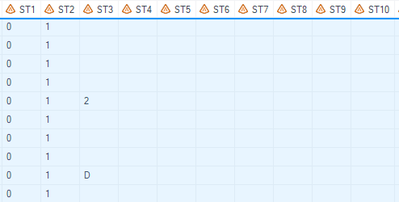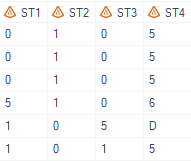## How to check for an array of columns and search for specific pattern of events

Hello folks, I think I've figured partially out about this issue at hand and it should be straightforward.

Basically, I want to search for values in an array of 21 columns and make sure I capture rows when event A occurs BEFORE event B. In my case, status 1 occurs before status 0, but not necessarily in adjacent columns. Below is what my data looks like:

each row is a unique ID, and I have column names from st1 till st21:I want to identify/signal the rows when value 1 occurs BEFORE value 0 per row and there could be other values in between the two values I want, but as long as 1 is before 0, I want to flag the row. Below is my code:

``````
DATA TEST; SET KEEP4;
ARRAY COLNAME{21} ST1-ST21;
FLG=0;
DO i=1 TO DIM(COLNAME);
IF COLNAME{i} ='1' THEN DO;
IF COLNAME{i+1}='0'
THEN DO;
FLG=1;
END;
END;
END;
DROP i;
RUN;

DATA TEST1; SET TEST;
WHERE FLG=1; RUN;``````

But the code above only selects the rows when 1 occurs RIGHT before 0 like below:There will be situations when 1 occurs, followed by a bunch of other values before 0 appears, how can I capture those?

Many thanks!

1 ACCEPTED SOLUTION

Accepted Solutions

## Re: How to check for an array of columns and search for specific pattern of events

To extend your current idea is easy.  You can take advantage of the power of the data step DO loop to make it even simpler.

``````data test1;
set have;
array colname st1-st21;
flg=0;
do i=1 to dim(colname) until (colname[i] ='1');
end;
do i=i+1 to dim(colname) until (flg);
flg=colname[i] ='0';
end;
if flg;
run;
``````

Or you can eliminate the first loop by using the WHICHC() function to find the first '1'.

``````data test1;
set have;
array colname st1-st21;
flg=0;
i=whichc('1', of colname[*]);;
if i then do i=i+1 to dim(colname) until (flg);
flg=colname[i] ='0';
end;
if flg;
run;``````

And if you don't want to include cases where there is a '0' before the first '1' then you could eliminate the do loops completely by just testing if the first '0' is after the first '1'.

``````data test1;
set have;
array colname st1-st21;
flg= 0 < whichc('1',of colname[*]) < whichc('0',of colname[*]);
if flg;
run;``````

4 REPLIES 4

## Re: How to check for an array of columns and search for specific pattern of events

It's a lot easier without arrays. Just create a string from the columns and search for a '1' followed by a '0':

``````data Have;
input st1 \$ st2 \$ st3 \$ st4 \$;
String = cats(of st1 - st4);
Flag = (findc(string,'1') and findc(string,'0', ,findc(string,'1')));
datalines;
0 1 5 0
0 1 0 5
0 1 0 5
5 1 0 6
1 0 5 D
1 0 1 5
1 2 1 1
;
run;``````

## Re: How to check for an array of columns and search for specific pattern of events

If the only condition is that there needs to be a sequence where 1 appears prior to 0 then below should work.

``````data Have;
input st1 \$ st2 \$ st3 \$ st4 \$;
flag = find(compress(cats(of st:),'01','k'),'10')>0;
datalines;
0 1 5 0
0 1 0 5
0 1 0 5
5 1 0 6
1 0 5 D
1 1 5 0
1 2 1 1
;
run;``````

With an array something like below should work.

``````data demo;
input st1 \$ st2 \$ st3 \$ st4 \$;
array _st {*} st:;
do _i=1 to dim(_st);
if _st[_i]='1' then flag=0;
else if flag=0 and _st[_i]='0' then
do;
flag=1;
leave;
end;
end;
if missing(flag) then flag=0;
drop _:;
datalines;
0 1 5 0
0 1 0 5
0 1 0 5
5 1 0 6
1 0 5 D
1 1 5 0
1 2 1 1
;
run;``````

## Re: How to check for an array of columns and search for specific pattern of events

To extend your current idea is easy.  You can take advantage of the power of the data step DO loop to make it even simpler.

``````data test1;
set have;
array colname st1-st21;
flg=0;
do i=1 to dim(colname) until (colname[i] ='1');
end;
do i=i+1 to dim(colname) until (flg);
flg=colname[i] ='0';
end;
if flg;
run;
``````

Or you can eliminate the first loop by using the WHICHC() function to find the first '1'.

``````data test1;
set have;
array colname st1-st21;
flg=0;
i=whichc('1', of colname[*]);;
if i then do i=i+1 to dim(colname) until (flg);
flg=colname[i] ='0';
end;
if flg;
run;``````

And if you don't want to include cases where there is a '0' before the first '1' then you could eliminate the do loops completely by just testing if the first '0' is after the first '1'.

``````data test1;
set have;
array colname st1-st21;
flg= 0 < whichc('1',of colname[*]) < whichc('0',of colname[*]);
if flg;
run;``````

## Re: How to check for an array of columns and search for specific pattern of events

Thanks @Tom ! this is exactly what I need! Much appreciated!

Discussion stats
• 4 replies
• 298 views
• 4 likes
• 4 in conversation